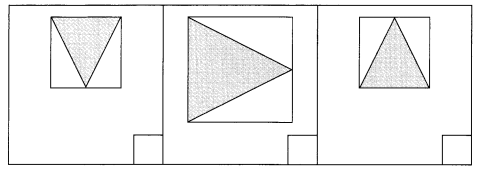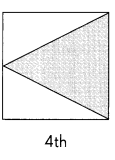# Math in Focus Grade 3 Chapter 18 Answer Key Two-Dimensional Shapes

This handy Math in Focus Grade 3 Workbook Answer Key Chapter 18 Two-Dimensional Shapes provides detailed solutions for the textbook questions.

## Math in Focus Grade 3 Chapter 18 Answer Key Two-Dimensional Shapes

Challenging Practice

Question 1.
Copy the figure on a dot paper. Cut it out and rearrange the shapes in the figure to form a square.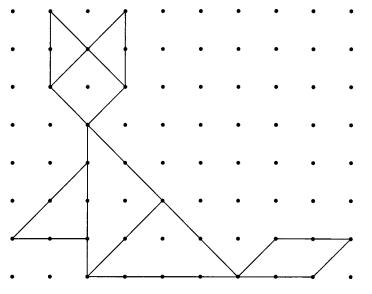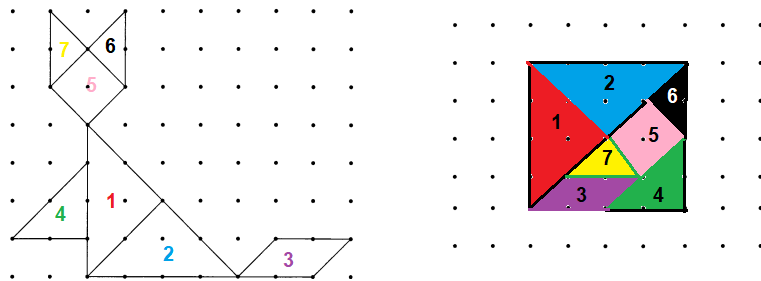Explanation:
Cut the given figure for re arrange, and color it as shown in the above picture
place big triangles as number 1 & 2 colored red and blue, now half of your figure is completed
now for second half of place 3 at any one corner such that you can easily fix red small pictures
A Square is a regular quadrilateral or polygon.
which has all the four sides of equal length, four vertices and,
four angles with right angle triangles.

Question 2.
Study the pattern to find the rule. Then draw the missing shape.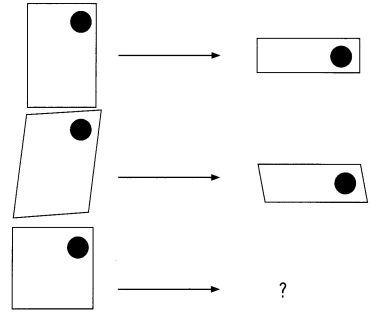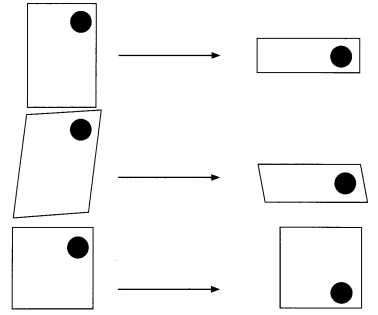Explanation:
The pattern is Turn or Rotation.
A turn or a rotation describes the motion of turning a shape as if it were drawn on a piece of paper.
The usual primary-grades vocabulary is a “turn” and the usual middle-grades vocabulary is “rotation”.

Problem Solving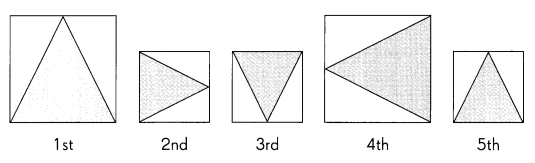Question 1.
Look at the repeated pattern.
a. Check the box that shows the next shape.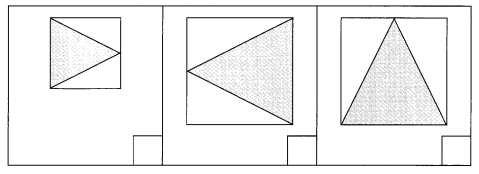3rd pattern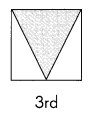Explanation:
The image is rotated in clockwise direction from horizontal axis to vertical axis,
such that the image rotates clockwise and the image resembles the same.

b. Check the box that shows the tenth shape.